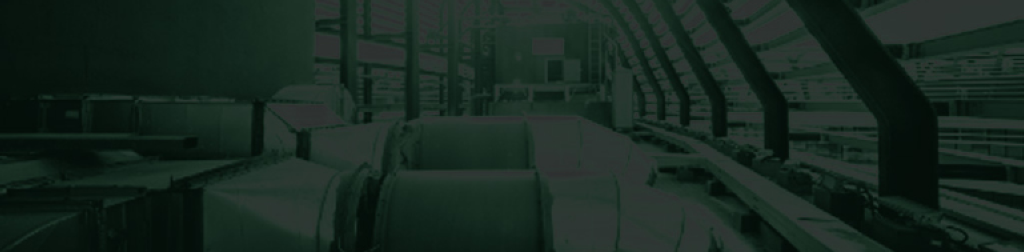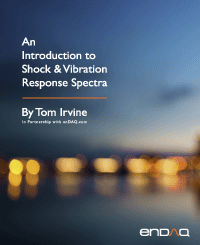Sample Rate & Aliasing
By Tom Irvine
SECTION 1

# Data Collection Accuracy

The wagon-wheel effect, or stroboscopic effect, is an optical illusion in which a spoked wheel appears to rotate at a different speed than its true rotation. The wheel can appear to rotate more slowly than the true rotation, appear stationary, or even appear to rotate in the opposite direction from the true rotation. The wagon-wheel effect is seen in film or television depictions of stagecoaches or wagons in Western movies, but the effect may also be seen in videos of helicopter rotors and aircraft propellers.

This optical illusion is a form of aliasing. It can occur in a video recording where the frame rate is too slow relative to the rotational or oscillation frequency of the moving object. A similar problem can occur when accelerometer data is digitized unless precautions are taken.

Engineers collect accelerometer data in a variety of settings both in the field and in lab tests. The accelerometers measure the data in analog form. The accelerometer may have an integral mechanical lowpass filter. Furthermore, the signal conditioning unit may have an analog lowpass filter. Lowpass filtering of the analog signal is necessary to prevent aliasing. Eventually, the data is passed through an analog-to-digital converter.

The proper lowpass frequency and sampling rate must be selected to ensure that the digitized data is accurate. There are two rules-of-thumb which must each be met.

SECTION 2
First Requirement

The first requirement is that the sampling rate must be greater than the maximum analysis frequency. Industry guidelines are summarized in the following table, where

 Analysis Type N Frequency Domain 2 Time Domain 10

The frequency domain requirement is based on the need for at least two time-domain coordinates per cycle to resolve a sine wave. The frequency domain analysis thus extends up to the Nyquist frequency which is one-half the sample rate. Note that some conservative references specify an N of 2.5 for frequency domain calculation.

A sampling rate of 100 kHz is thus required for a shock response spectrum (SRS) analysis extending to 10 kHz. The SRS function is covered in Section 0. Recall that the SRS is calculated in the time domain.

The IES Handbook for Dynamic Data Acquisition and Analysis  gives the following guidelines:

Unlike other spectral quantities evolving from the discrete Fourier transform computations, the SRS is essentially a time domain quantity. Hence, the digital sampling rate given by Rs=1/Δt introduces errors beyond those associated with aliasing about the Nyquist frequency. Thus, Rs must be high enough to accurately describe the response of the SRS oscillators. To minimize potential error, it is recommended that the SRS computations be performed with a sampling rate of Rs > 10 fh, where fh is the highest natural frequency of the SRS computation.

SECTION 3
Second Requirement

Shannon’s sampling theorem states that a sampled time signal must not contain components at frequencies above the Nyquist frequency.

Again, the Nyquist frequency is equal to one-half the sampling rate. Shannon's theorem applies to frequency domain analysis.

Thus, the second requirement is that the sampling rate must be greater than the maximum frequency present in the source energy at the measurement location. This requirement is independent of the maximum analysis frequency.

The guidelines are summarized in the following table, where

 Analysis Type M Frequency Domain 2 Time Domain 10

Note the similarity between Table 1.1 and Table 1.2.

SECTION 4
Anti-aliasing Filter

The challenge is that the maximum frequency in the source energy maybe unknown, which is the usual case for field data. The solution is to use an analog lowpass filter prior to digitization. This filter is also referred to as an anti-aliasing filter. The filter cut-off frequency is typically set at, or slightly above, the maximum analysis frequency.

The IES Handbook for Dynamic Data Acquisition and Analysis gives the following guidelines.

Let

fC be the cutoff frequency
fN be the Nyquist frequency

• A lowpass anti-aliasing filter with a cutoff rate of at least 60 dB/octave should be used for the analog-to-digital conversion of all dynamic data.
• With a 60 dB/octave cutoff rate, the half-power point cutoff frequency of the filter should be set at fc < 0.6 fN.
• If the anti-aliasing filter has a more rapid cutoff rate, a higher cutoff frequency can be used, but the bound fc < 0.8 fN should never be exceeded.

Mild detonating cord is source shock and is coiled on the underside of the plate. Accelerometers are mounted on the plate to record the shock level. The component is powered and monitored during the test. The source shock may have energy components exceeding 100 kHz. Use of an analog anti-aliasing filter is critical. Otherwise, high frequency energy will be erroneously folded down to lower frequencies in the shock response spectrum.

SECTION 5
Aliasing Example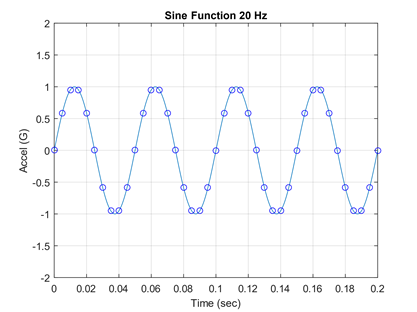Figure 5.1. A 20 Hz Sine Function Sampled at 200 Hz

There are 10 points per period. The Nyquist frequency is 100 Hz.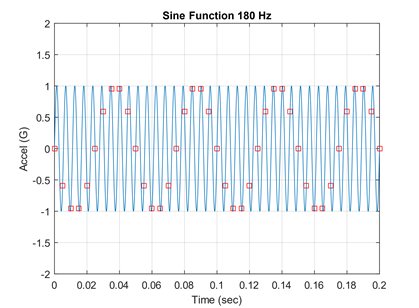Figure 5.2. An 1800 Hz Sine Function Samples at 2000 Hz

The red markers show that there are 1.11 points per 1800 Hz cycle. The Nyquist frequency is 1000 Hz. Aliasing occurs!

The two signals both appear as 200 Hz although only one truly is.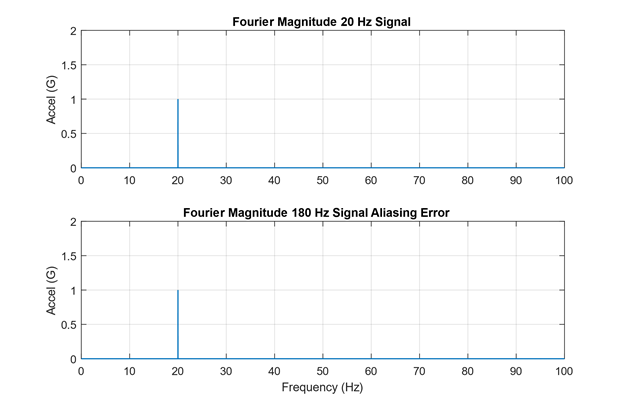Figure 5.4. Fourier Magnitude of Each Signal Sampled at 200 Hz

The 180 Hz signal is folded about the Nyquist frequency which is 100 Hz. The resulting energy is deposited at 20 Hz. Fourier transforms are covered in Section 15.

As an example, consider a pyrotechnic shock test with a time domain maximum analysis frequency of 10 kHz. The sample rate should be at least 100 kHz. The Nyquist frequency is 50 Hz. Assume a lowpass filter with a 60 dB/octave cutoff rate. The maximum cutoff frequency should thus be 30 kHz. These are values to satisfy basic requirements. A better approach would be to set the sample rate at 250 kHz or more if the measurement is going to be in the “near field” relative to the source. This higher sample rate can be readily achieved in a ground test, but not in flight where the telemetry bandwidth is limited.

As another example, consider a vibration test with a maximum analysis frequency of 2 kHz in the time domain. The sample rate should be at least 20 kHz. The Nyquist frequency is 10 kHz. The maximum cutoff frequency should thus be 6 kHz.